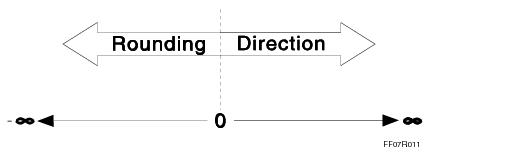# 15.10 - Rounding - Teradata Database

## Teradata Database SQL Data Types and Literals

prodname
vrm_release
15.10
category
Programming Reference
featnum
B035-1143-151K

If a value being inserted does not fit into a DECIMAL or NUMERIC column, the value is rounded. Teradata Database rounds using the digit to the right of the rounding digit, the last digit that fits into the DECIMAL/NUMERIC field.

 IF the value of the digit to the right of the rounding digit is … THEN the value of the rounding digit … < 5 does not change. > 5 increases by one.

Additional considerations come into play when the value of the digit to the right of the rounding digit is exactly five. One consideration is the value of the DBS Control Record RoundHalfwayMagUp field.

When the DBS Control Record RoundHalfwayMagUp field is set to FALSE, then rounding is performed as explained in the following table. This is the default.

 IF the value of the digit to the right of the rounding digit is exactly 5 and … THEN ... there are no trailing nonzero digits If the value of the rounding digit is odd, then the value of the rounding digit increases by one. If the value of the rounding digit is even, then the value of the rounding digit does not change. there are trailing nonzero digits rounding behaves as if the value of the digit to the right of the rounding digit is greater than five.

The following table shows the results of inserting values into a DECIMAL(3,2) column.

 WHEN the value of the INSERT is … THEN the value is rounded … Because the value of the digit to the right of the rounding digit is … AND the result is … .014 down < 5 .01 .015 up 5 and last stored position is odd .02 .0151 up > 5 .02 .024 down < 5 .02 .025 down 5 and last stored position is even. .02 .0251 up > 5 .03

When the DBS Control Record RoundHalfwayMagUp field is set to TRUE, then the magnitude of all halfway values, both negative and positive, is rounded up, away from zero (see the illustration).While this is the most common rounding semantics for business applications, it is also nontrivially biased in the upward direction.

For additional information about the DBS Control Record and the RoundHalfwayMagUp field, see Utilities.

Conversion to FLOAT/REAL/DOUBLE PRECISION rounds to the nearest value available. Neither decimal fractions nor numbers greater than 9,007,199,254,740,992 can be guaranteed to be represented exactly, so the nearest representable value is chosen. If there are two representable values that qualify as the nearest value, then the representation with a '0' in the least significant bit is chosen.

For example, 0.1, when stored in a FLOAT column, is rounded to a value slightly higher: 0.1000000000000000055511151231257827021181583404541015625.

When rounding a value to NUMBER type, the nearest NUMBER to the value is chosen, taking into account the NUMBER scale. If there are two NUMBER values the same distance from the value, the rounding rule is determined by the DBS Control field RoundNumberAsDec. By default, the RoundNumberAsDec field is FALSE, which indicates that the digit will be rounded up, away from zero. If you want NUMBER rounding to follow DECIMAL rounding behaviour, set the RoundNumberAsDec field to TRUE, in which case the rounding rule will be determined by the DBS Control field RoundHalfwayMagUp.

For additional information about the DBS Control Record, and the RoundNumberAsDec and RoundHalfwayMagUp fields, see Utilities.